Q.1. Find the loss of the electrical potential energy when two charged capactiors are connected in parallel

Ans ⇒ Let the two capacitors have capacity potential, and charge respectively C1, V1, Q1, and C2, V2 and Q2 and before joining them their energy.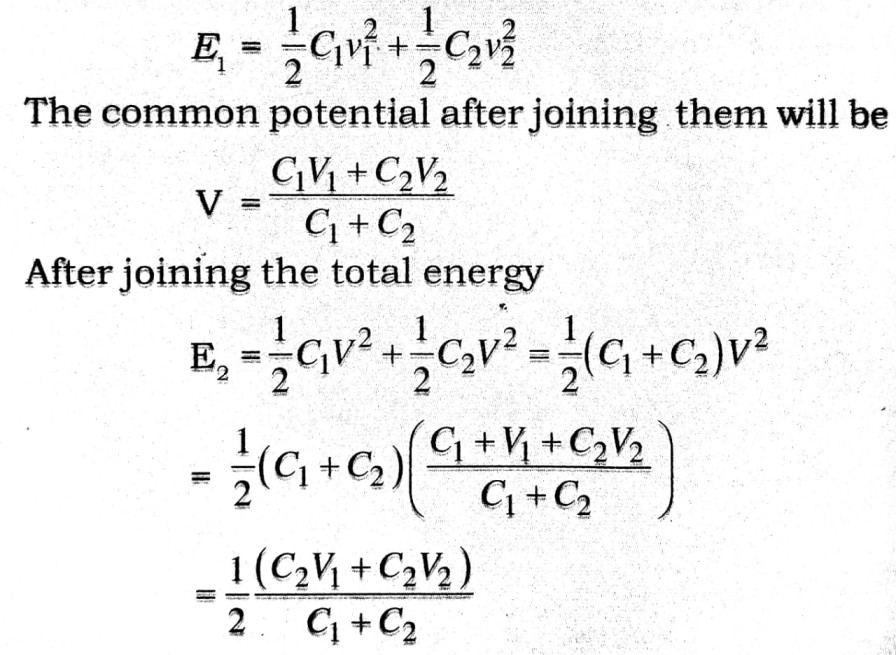Loss of energy = E1 – E2
It is a positive quanlty because E1 > E2 hence there is a loss of energy.

Q.2. Define capacitance and write its S.I. unit. Show that energy stored in a parallel plate capacitor is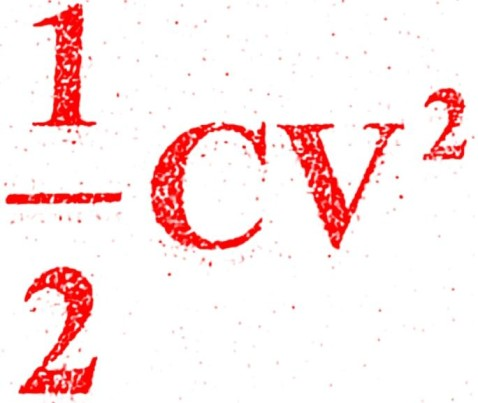. Hence find an expression on for energy density.

Ans ⇒ The capacitance of an isolated conductor is the ratio of charge on the conductor to its electric potential. If a charge q be placed on a conductor, its potential V bears a fixed ratio with the charge Q, so that q/v is a constant, This ratio is called its capacitance C.
C = q/v
SI unit : Farad is the S.I unit of capacitance which is equal to 1 coulomb per volt.

Energy stored in a parallel plate capcitor : Let q be the charge on a parallel plate capacitor, Then potential difference between the plates V will be given by V2 – V1 = q/c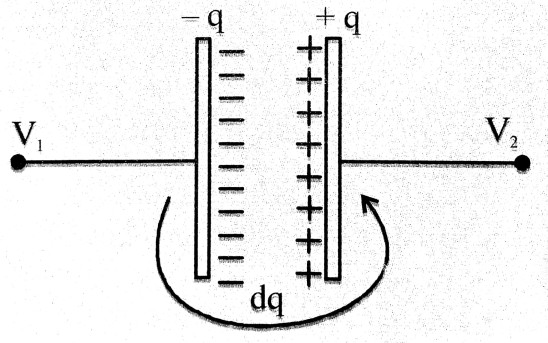Let a charge dq, be transferred from negative to positive plate. The initial energy of this charge is dqV1 and final energy is dqV2. Workdone on the charge is stored in the capacitor and equals
dU = dqV2 – dqV1 = dq (V2– V1) = dqV                               …(ii)
From (i) and (ii)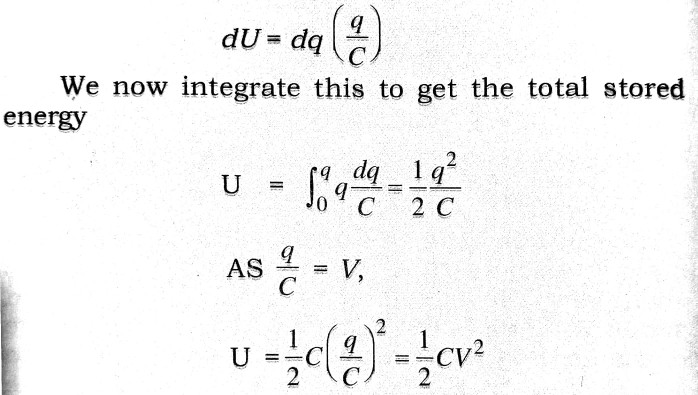This is the required expression.
Energy Density : The energy stored per unit volume of the space (spanned by electric field) is the energy density. Let a parallel plate capacitor have a plate area S and separation D. Then volume occupied by field is Sd. Its capacitance C is given by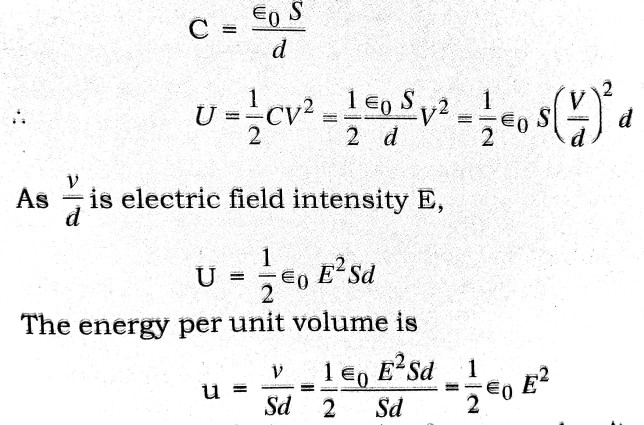This is the required expression for energy density.

Q.3. Derive an expression for the capacitance of a parallel plate capacitor. If a compound dielectric medium is introduced between the plates of the capacitor, how will the capacitance change ?

Ans ⇒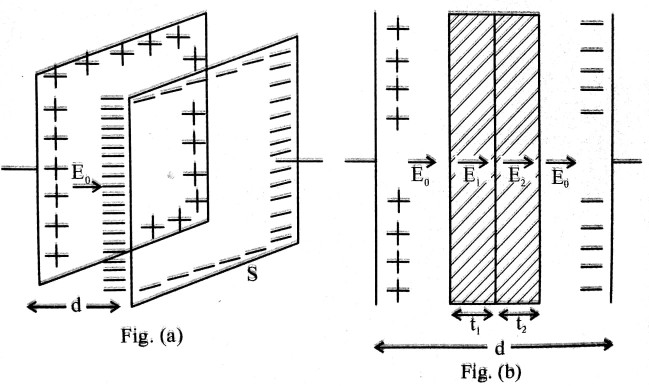The figure (a) shows a parallel plate capacitor with out dielectric. The plates each of area S, are separated by a distance d. Let Q be the charge. Then electric field intensity E0 is given by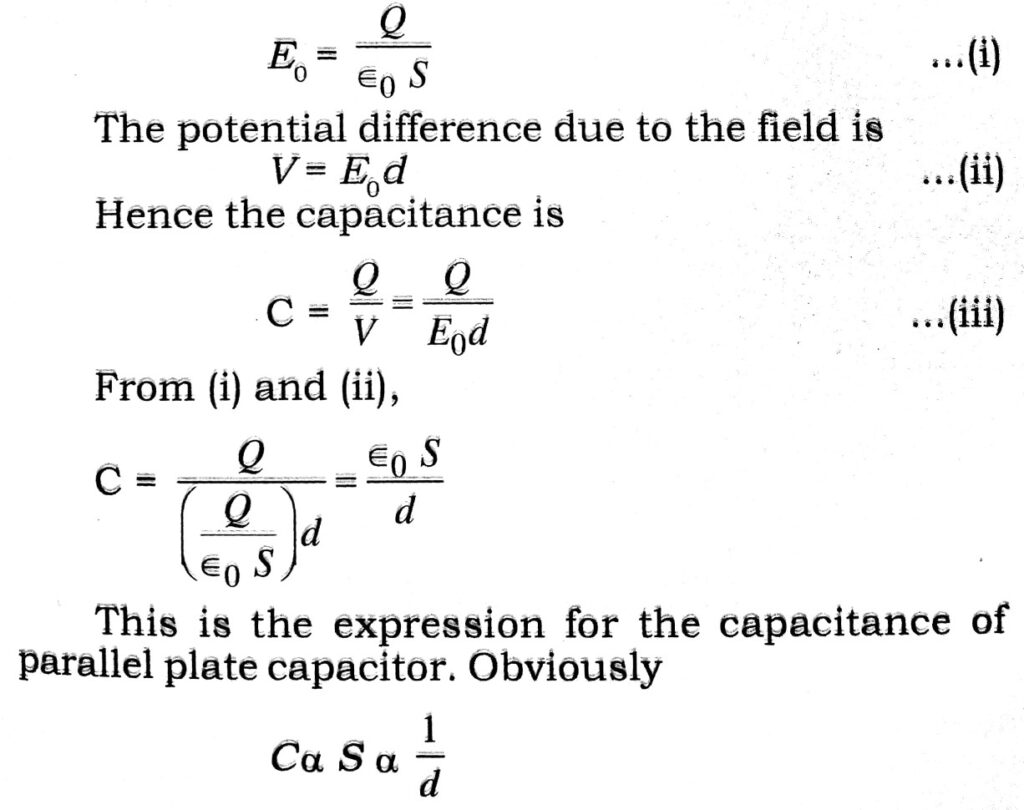Effect of dielectric : In the figure (b) compound slab of two dielectrice is Introduced. The fields are now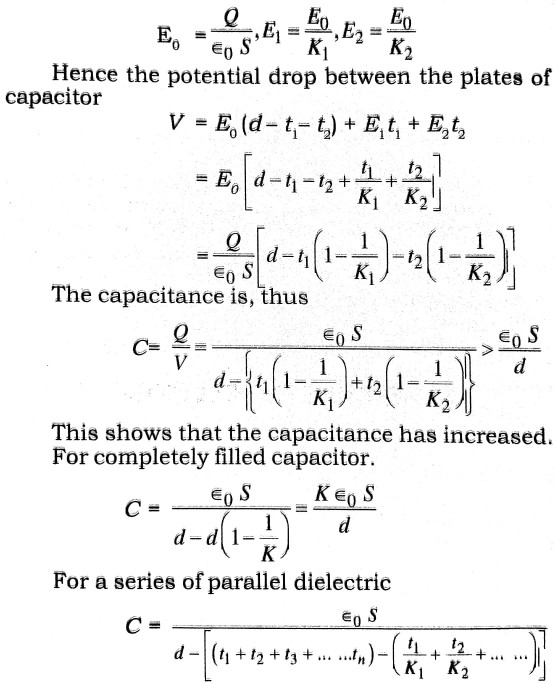The effect of dielectric is to increase the capacitance.

Q.4. Giving a labelled diagram, explain the principle and working of a Van de Graph generator.

Ans ⇒ Van de Graaf generator is a device capable of producing potential differences of a few million volts and fields close to the breakdown field of air which is about 3 x 106 V/m. It is used to accelerate charged particles to high speeds.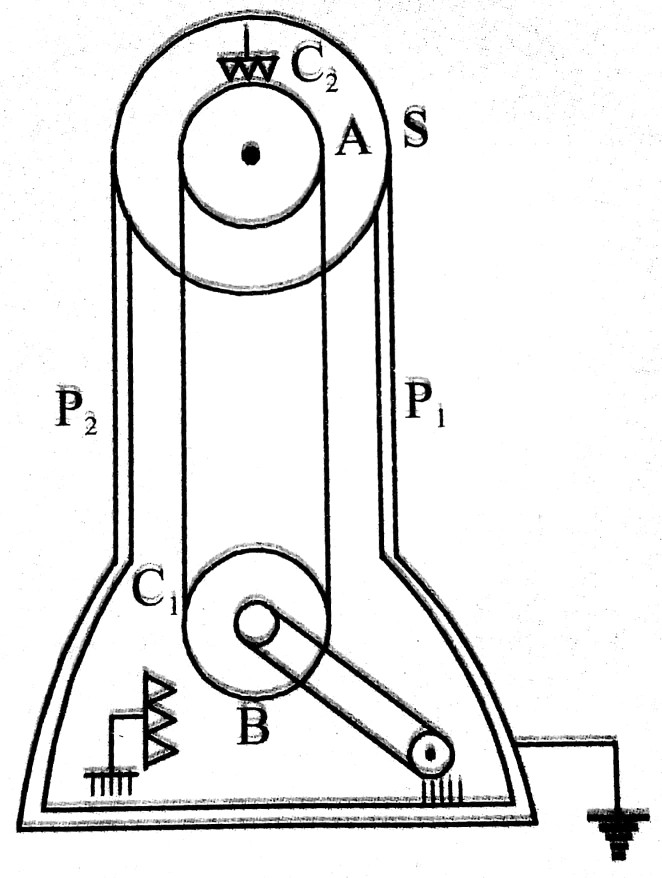Principle : The working of Van de Graaff of Van de Graaf generator is based on the following two electrostatic phenomena.

(i) Electric fields and surface charge densities tend to have lower values on the smooth parts of the surface of a conductor whereas shape points and corners tend to have high charge densities and fields.

(ii) An electric charge given to a conductor is distributed entirely on its outer surface.

Construction and Working : Fig. shows a schematic diagram of a Van de Graaff generator. A large conducting spherical shell having radius of a few metres, is supported at a height of several metres above the ground on an insulating column. A long, narrow belt of some insulating material is wound around two pulleys, and at the ground level and the other at the centre of the shell. The belt is kept continuously moving by a motor driving the lower pulley. It continuously carries positive charge, sprayed on to it by a metal brush, to the top. There it transfers this charge to another metal brush connected to the large shell. Thus, positive charge is transferred to the shell where it spreads out uniformly on the outer surface. In this way high voltage can be built up.

## Class 12th physics Long Type question in English

 S.N Physics Long Type Question English Medium 1. ELECTRIC CHARGES AND FIELDS 2. LECTROSTATIC POTENTIAL AND CAPACITANCE 3. 4. 5. MAGNETISM AND MATTER 6. ELECTROMAGNETIC INDUCTION 7. ALTERNATING CURRENT 8. ELECTROMAGNETIC WAVES 9. RAY OPTICS AND OPTICAL INSTRUMENTS 10. WAVE OPTICS 11. DUAL NATURE OF MATTER AND RADIATION 12. ATOMS 13. NUCLEI 14. SEMI CONDUCTOR ELECTRONICS 15. COMMUNICATION SYSTEMS